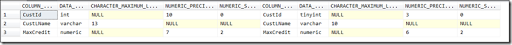## Wednesday, 9 March 2016

### String or binary data would be truncated / Arithmetic overflow error converting numeric to data type numeric – Workaround

There’s nothing more annoying than getting the error ‘String or binary data would be truncated’ or ‘Arithmetic overflow error converting numeric to data type numeric’, when you need to insert data to a table using a SELECT statement. To make it more interesting, the SQL won’t be providing us the name of the column (or columns) which is causing this issue. (This is due to the SQL architecture on how it executes queries)

To illustrate this I will use a small sample.

Suppose we have a table to store some Customer details:

CREATE TABLE Customer_Data(
CustId        TINYINT
,CustFName    VARCHAR(10)
,CustLName    VARCHAR(10)
,MaxCredit    NUMERIC(6,2)
)

We will try to insert details to the above table. (In reality the SELECT statement will be very complex and could fetch lots of rows)

INSERT INTO dbo.Customer_Data(
CustId
,CustFName
,CustLName
,MaxCredit
)

SELECT 1 AS CustId,'John' AS CustFName,'Doe' AS CustLName,1000.00 AS MaxCredit UNION ALL
SELECT 2,'Jane','Doe',1000.00 UNION ALL
SELECT 3,'James','Whitacker Jr.',15000.00

This will result the following error:

Msg 8152, Level 16, State 14, Line 48
String or binary data would be truncated.

The statement has been terminated.

The challenge here is to find out actually which columns are having this issue. (As mentioned in reality number of columns could be very large)

However there is a small workaround which we can use to find out the columns which is causing the insertion to fail. You need to do the following in order to find out these columns.

1. First create a table using the same select statement. (You can either create a temporary table or an actual table based on the environment and your need). I will create two tables, one actual and one temporary to illustrate both the options.

SELECT A.*
INTO Temp_Customer_Data
FROM(
SELECT 1 AS CustId,'John' AS CustFName,'Doe' AS CustLName,1000.00 AS MaxCredit UNION ALL
SELECT 2,'Jane','Doe',1000.00 UNION ALL
SELECT 3,'James','Whitacker Jr.',15000.00
) AS A

SELECT A.*
INTO #Customer_Data
FROM(
SELECT 1 AS CustId,'John' AS CustFName,'Doe' AS CustLName,1000.00 AS MaxCredit UNION ALL
SELECT 2,'Jane','Doe',1000.00 UNION ALL
SELECT 3,'James','Whitacker Jr.',15000.00
) AS A

2. Use the following query to identify the issue columns

Actual Table:

;WITH Cte_Source AS (
SELECT
C.COLUMN_NAME
,C.DATA_TYPE
,C.CHARACTER_MAXIMUM_LENGTH
,C.NUMERIC_PRECISION
,C.NUMERIC_SCALE
FROM
INFORMATION_SCHEMA.TABLES AS T
JOIN INFORMATION_SCHEMA.COLUMNS AS C
ON C.TABLE_CATALOG = T.TABLE_CATALOG
AND C.TABLE_NAME = T.TABLE_NAME
AND C.TABLE_SCHEMA = T.TABLE_SCHEMA
WHERE
T.TABLE_NAME = 'Temp_Customer_Data'        -- Source Table
AND T.TABLE_SCHEMA = 'dbo'
)
,Cte_Destination AS (
SELECT
C.COLUMN_NAME
,C.DATA_TYPE
,C.CHARACTER_MAXIMUM_LENGTH
,C.NUMERIC_PRECISION
,C.NUMERIC_SCALE
FROM
INFORMATION_SCHEMA.TABLES AS T
JOIN INFORMATION_SCHEMA.COLUMNS AS C
ON C.TABLE_CATALOG = T.TABLE_CATALOG
AND C.TABLE_NAME = T.TABLE_NAME
AND C.TABLE_SCHEMA = T.TABLE_SCHEMA
WHERE
T.TABLE_NAME = 'Customer_Data'        -- Destination Table
AND T.TABLE_SCHEMA = 'dbo'
)
SELECT
S.COLUMN_NAME
,S.DATA_TYPE
,S.CHARACTER_MAXIMUM_LENGTH
,S.NUMERIC_PRECISION
,S.NUMERIC_SCALE

,D.COLUMN_NAME
,D.DATA_TYPE
,D.CHARACTER_MAXIMUM_LENGTH
,D.NUMERIC_PRECISION
,D.NUMERIC_SCALE
FROM
Cte_Source AS S
JOIN Cte_Destination AS D
ON D.COLUMN_NAME = S.COLUMN_NAME
WHERE
S.CHARACTER_MAXIMUM_LENGTH > D.CHARACTER_MAXIMUM_LENGTH
OR S.NUMERIC_PRECISION > D.NUMERIC_PRECISION

Temporary Table:

;WITH Cte_Source AS (
SELECT
C.COLUMN_NAME
,C.DATA_TYPE
,C.CHARACTER_MAXIMUM_LENGTH
,C.NUMERIC_PRECISION
,C.NUMERIC_SCALE
FROM
tempdb.sys.objects so
JOIN tempdb.INFORMATION_SCHEMA.TABLES AS T
ON so.name = T.TABLE_NAME
AND so.[object_id] = OBJECT_ID('tempdb..#Customer_Data')
JOIN tempdb.INFORMATION_SCHEMA.COLUMNS AS C
ON C.TABLE_CATALOG = T.TABLE_CATALOG
AND C.TABLE_NAME = T.TABLE_NAME
AND C.TABLE_SCHEMA = T.TABLE_SCHEMA

WHERE
T.TABLE_SCHEMA = 'dbo'
)
,Cte_Destination AS (
SELECT
C.COLUMN_NAME
,C.DATA_TYPE
,C.CHARACTER_MAXIMUM_LENGTH
,C.NUMERIC_PRECISION
,C.NUMERIC_SCALE
FROM
INFORMATION_SCHEMA.TABLES AS T
JOIN INFORMATION_SCHEMA.COLUMNS AS C
ON C.TABLE_CATALOG = T.TABLE_CATALOG
AND C.TABLE_NAME = T.TABLE_NAME
AND C.TABLE_SCHEMA = T.TABLE_SCHEMA
WHERE
T.TABLE_NAME = 'Customer_Data'        -- Destination Table
AND T.TABLE_SCHEMA = 'dbo'
)
SELECT
S.COLUMN_NAME
,S.DATA_TYPE
,S.CHARACTER_MAXIMUM_LENGTH
,S.NUMERIC_PRECISION
,S.NUMERIC_SCALE

,D.COLUMN_NAME
,D.DATA_TYPE
,D.CHARACTER_MAXIMUM_LENGTH
,D.NUMERIC_PRECISION
,D.NUMERIC_SCALE
FROM
Cte_Source AS S
JOIN Cte_Destination AS D
ON D.COLUMN_NAME = S.COLUMN_NAME
WHERE
S.CHARACTER_MAXIMUM_LENGTH > D.CHARACTER_MAXIMUM_LENGTH
OR S.NUMERIC_PRECISION > D.NUMERIC_PRECISION

Both the aforementioned queries will return the following result.The reason to return the above three columns as follows:

1. CustId ==> In our destination table CustId’s data type is TINYINT. Even the select query is returning the results within the boundary, the data type which our insertion query is returning is an INT. So there could be a possibility that there could be large numbers that the destination table could not hold.

2. CustName ==> ‘Whitacker Jr.’ is exceeding the maximum length of 10 which is in the destination table.

3. MaxCredit ==> In the destination table the size of the column is numeric (6,2). Which means it can hold values up to 9999.99. But our insertion query contains a record which consists of 15000.00.

Hope this might be helpful to you.

## Thursday, 3 March 2016

### Extracting Date (Excluding Time) from a DateTime value in SQL Server

SQL Server supports many data types where we can store the Date along with the time, such as

• DateTime
• SmallDateTime
• DateTimeOffset
• DateTime2

But in some cases it’s required only to fetch only the date portion from an aforementioned type of field.

There are few ways which we can achieve this task easily using T-SQL.

The easiest of the method is to CAST the DateTime value directly to a DATE type.

SELECT CAST(GETDATE() AS DATE)                                    --==> 2016-03-03

Also you can achieve this by using the CONVERT function providing different styles as per your requirement.

SELECT CONVERT(VARCHAR(24),GETDATE(),101)                        --==> 03/03/2016
SELECT CONVERT(VARCHAR(24),GETDATE(),102)                        --==> 2016.03.03

Please refer to the following URL (https://msdn.microsoft.com/en-sg/library/ms187928.aspx) for more details on the CONVERT function and supported styles:

But if your requirement is to return a DateTime type but having only the date portion you can use the following syntax:

SELECT CAST(FLOOR(CAST(GETDATE() AS FLOAT)) AS DATETIME)        --==> 2016-03-03 00:00:00.000
SELECT CAST(CONVERT(VARCHAR(8),GETDATE(),112) AS DATETIME)      --==> 2016-03-03 00:00:00.000